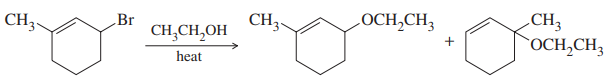×
Get Full Access to Organic Chemistry - 8 Edition - Chapter 15 - Problem 5p
Get Full Access to Organic Chemistry - 8 Edition - Chapter 15 - Problem 5p

×

# When 3-bromo-1-methylcyclohexene undergoes solvolysis inISBN: 9780321768414 33

## Solution for problem 5P Chapter 15

Organic Chemistry | 8th Edition

• Textbook Solutions
• 2901 Step-by-step solutions solved by professors and subject experts
• Get 24/7 help from StudySoup virtual teaching assistantsOrganic Chemistry | 8th Edition

4 5 1 364 Reviews
27
2
Problem 5P

When 3-bromo-1-methylcyclohexene undergoes solvolysis in hot ethanol, two products are formed. Propose a mechanism that accounts for both of these products.Equation Transcription:Text Transcription:

CH_3

Br

CH_3CH_2OH

CH_3

OCH_2CH_3

CH_3

OCH_2CH_3

Step-by-Step Solution:
Step 1 of 3

Solution 5PThe two resonance allylic carbocation reacts with nucleophile ethanol to form two products.

Step 2 of 3

Step 3 of 3

##### ISBN: 9780321768414

This full solution covers the following key subjects: methylcyclohexene, products, mechanism, ethanol, formed. This expansive textbook survival guide covers 25 chapters, and 1336 solutions. This textbook survival guide was created for the textbook: Organic Chemistry, edition: 8. The full step-by-step solution to problem: 5P from chapter: 15 was answered by , our top Chemistry solution expert on 05/06/17, 06:41PM. The answer to “?When 3-bromo-1-methylcyclohexene undergoes solvolysis in hot ethanol, two products are formed. Propose a mechanism that accounts for both of these products. Equation Transcription:Text Transcription:CH_3BrCH_3CH_2OHCH_3OCH_2CH_3CH_3OCH_2CH_3” is broken down into a number of easy to follow steps, and 24 words. Since the solution to 5P from 15 chapter was answered, more than 829 students have viewed the full step-by-step answer. Organic Chemistry was written by and is associated to the ISBN: 9780321768414.

## Discover and learn what students are asking

Calculus: Early Transcendental Functions : Conics, Parametric Equations, and Polar Coordinates
?In Exercises 1–6, match the equation with the correct graph. [The graphs are labeled (a), (b), (c), (d), (e), and (f).]

Calculus: Early Transcendental Functions : First-Order Linear Differential Equations
?In Exercises 5-14, solve the first-order linear differential equation. $$\frac{d y}{d x}+\left(\frac{1}{x}\right) y=6 x+2$$

Calculus: Early Transcendental Functions : Partial Derivatives
?In Exercises 7 - 38, find both first partial derivatives. $$z=x^{2} e^{2 y}$$

Statistics: Informed Decisions Using Data : Measures of Position and Outliers
?Explain the advantage of using z-scores to compare observations from two different data sets.

Unlock Textbook Solution